# Completely multiplicative function

## Definition

Supposeis an arithmetic function. In other words,is a function from the natural numbers to some commutative unital ring. Then,is termed completely multiplicative or totally multiplicative if it is a monoid homomorphism from the multiplicative monoid of natural numbers to the multiplicative monoid of. In other words,satisfies the following two conditions:

•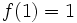.
•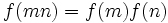for all natural numbers. Here, the multiplication on the left happens in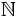while the multiplication on the right happens in.

## Facts

### Determined by values at primes

A completely multiplicative function is completely determined by the values it takes at all prime numbers. Further, there is no interdependence between the values taken at prime numbers. In other words, completely multiplicative functions correspond to infinite sequences of elements of the ring: given a sequence, the corresponding completely multiplicative function is the function sending theprime to theelement of the sequence.

### Dirichlet series

Further information: Product formula for Dirichlet series of completely multiplicative function

The Dirichlet series of a completely multiplicative function can be expressed particularly nicely in terms of the values at primes. Formally, ifis completely multiplicative, then: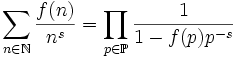,

where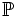is the set of primes.

## Effect of operations

### Dirichlet product

The Dirichlet product of completely multiplicative functions need not be completely multiplicative. However, it is a multiplicative function. Further information: Multiplicative functions form a group under Dirichlet product

### Pointwise product

A pointwise product of completely multiplicative functions is completely multiplicative.

### Composition

Ifis a completely multiplicative function with the property thattakes only positive integer values, andis a completely multiplicative function, thenmakes sense and is also completely multiplicative.